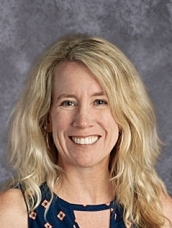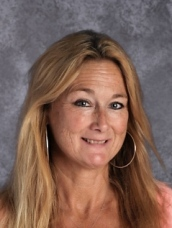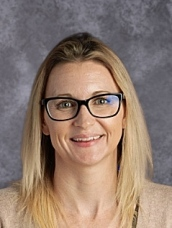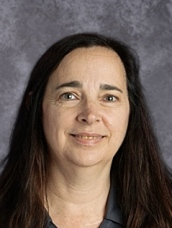Marsh Jr. High School - MathDeidra Anderson, Room D16
Math A
895 - 4110 x 316Greg Bishop, Room D14
Math A
895 - 4110 x 314Gina Hoffman, Room D15
IM1 Math
895 - 4110 x 315Victoria Leitner, Room D18
Math B & C
895 - 4110 x 318Robert Preston, Room C8
Math B & C
895 - 4110 x 308Julia Smith, Room D13
Math B & C
895 - 4110 x 313

## Course descriptions

In Math A, instructional time will focus on three critical areas:
1. Connecting ratio and rate to whole number multiplication and division, and using concepts of ratio and rate to solve problems
2. Developing fluency with fraction and decimal operations and how to convert between the two
3. Extending the notion of number to the system of rational numbers, which includes negative numbers.

In Math B, instructional time will focus on four critical areas:
1. Developing understanding of and applying proportional relationships
2. Developing understanding of operations with rational numbers and working with expressions and linear equations
3. Solving problems involving scale drawings and informal geometric constructions, and working with two- and three-dimensional shapes to solve problems involving area, surface area, and volume
4. Drawing inferences about populations based on samples.

.